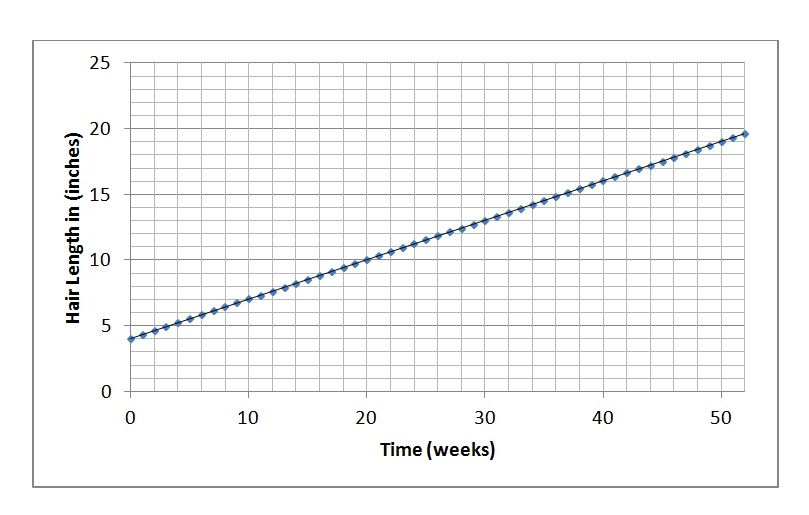# Hairy Questions

The next bunch of posts are intended for my incoming AP-C Physics students.   The course is a calculus-based version of 1st-year college level physics.  Some of my students will be starting to study calculus just as they are starting this course.  These posts are designed to give them enough of an introduction to stay above water.  So here we go…

Slope is a rate of change.

Many of the quantities you have already encountered in your study of physics are rates of change of other quantities. For example:

Velocity is the rate of change of position with respect to time.

Acceleration is the rate of change of velocity with respect to time.

Force is the rate of change of momentum with respect to time.

Current is the rate of change of electric charge with respect to time.

Induced Voltage is the rate of change of magnetic flux with respect to time.

And there were many times when you found the rate of change by graphing the quantity vs. time and then evaluating the slope of the resulting line.  Let’s look at a non-physics example.

For one year, you let your hair grow, measuring the length at the end of every week.  You graph the results of your experiment below, along with the line of best fit.There are some questions that we can answer by reading the information directly from this graph.  For example…

1. How long was your hair after week 10?

2. When did your hair reach a length of 15 inches?

3. How much longer did you hair grow between the end of week 20 and the end of week 30?

(Post a comment if you have answers or questions about any of these.)

Then there are questions that you answer by finding the slope.

4. What was the average rate of hair growth over the first 5 weeks?

5. What was the average rate of hair growth between week 10 and week 20?

6. What was the average rate of hair growth for the entire year?

7. At what rate was your hair growing at the end of week 30?

Questions 4, 5 and 6 are all answered the same way: you find the rise over run to determine the rate of change.  This should remind you of your first weeks in physics class, studying kinematics.  We could even call this slope  the “hair velocity” if we like.

And in fact, you should get the same answer to all three questions.  The graph is linear.  You will find the same slope no matter what two points you pick.  We can say that your hair is growing at constant velocity.

Question 7 is different from the others in a subtle but important way.  It asks you to find the growth rate at a specific time – and not over a time interval!  It is asking for an instantaneous rate of change, not an average.  Fortunately, this time, it’s still an easy question.  Since we have seen that the growth is at a constant rate, it is reasonable to assume that the rate at any particular instant is the same as the average rate for the entire period (or in fact, any period you pick!  It doesn’t matter – the rate is constant.)

But while that gives us an answer, it also lets us side-step some important questions:

1.  How would we find the rate of change at a given time if your hair growth rate wasn’t constant?

2.  What do we even mean by the rate AT a given time?  Don’t you need to compare TWO times in order to find a rate of change?

We’ll consider these in the nest post when you experiment with some hair-growth-acceleration tonic you found on line.

Comments are closed.